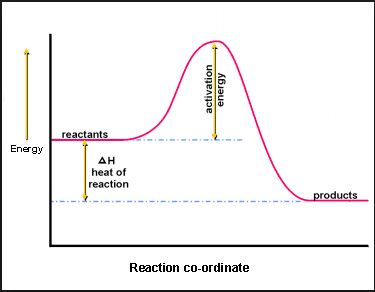# Thermodynamics and its Phenomenon

Thermodynamics deals with the concepts of heat and temperature and the inter-conversion of heat and other forms of energy. The four laws of thermodynamics govern the behavior of these quantities and provide a quantitative description.

Start Quiz

A sample of iron receives 50J of heat energy that raises the temperature of the iron by 25.0°C. If the iron has a specific heat of 0.10 J/g°C, what is the mass of the iron sample?

22 g

20 g

25 g

30 g

Determine the energy (in J) required to raise the temperature of 100.0 g of water from 20.0 C to 85.0 C? The specific heat of water is 4.184 J/g°C

250006 J

37196 J

27196 J

27200 J

Refer the the following question:
2N2 + 3H2 --> 2NH3 + 46 kJ
How much energy would be produced if only 1 mol of nitrogen was reacted?

92 kJ

23 KJ

22 KJ

43 KJ

True or False, The Law of Conservation of Energy states that energy cannot be created nor destroyed only transferred.

True

False

What mass of P4 must be reacted to produce 5905 kJ of energy?
P4 + 6Cl2 --> 4PCl3 + 2439 kJ

323g

783g

300g

231g

What kind of reaction is this?Exothermic

Endothermic

Ectothermic

None of these

Chemical reactions that absorb heat energy are called __________ and have a __________ enthalpy.

Exothermic, positive

Exothermic, negative

Endothermic, negative

Endothermic, positive

The study of heat energy that is involved in chemical and physical changes is _______.

Efficiency

Thermodynamics

Equilibrium

Energy transfer

Quiz/Test Summary
Title: Thermodynamics and its Phenomenon
Questions: 8
Contributed by: